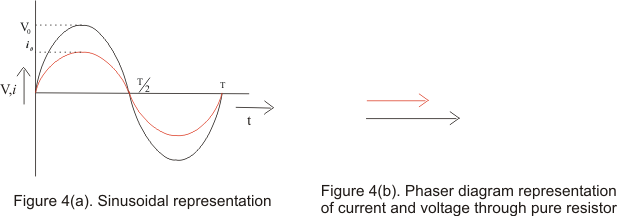# Phasor diagrams|Alternating Current|A.C through pure resistor

## (4) Root Mean square value of AC

• We know that time average value of AC over one cycle is zero and it can be proved easily
• Instantaneous current I and time average of AC over half cycle could be positive for one half cycle and negative for another half cycle but quantity i2 would always remain positive
• So time average of quantity i2 is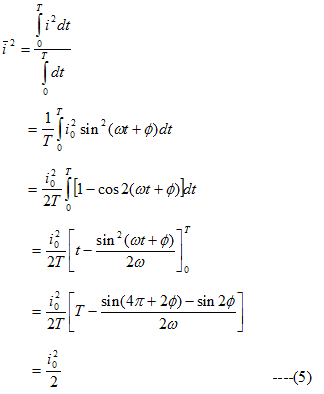This is known as the mean square current
• The square root of mean square current is called root mean square current or rms current.
Thus,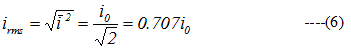thus ,the rms value of AC is .707i0 of the peak value of alternating current
• Similarly rms value of alternating voltage or emf is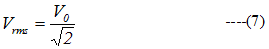• If we allow the AC current represented by i=i0sin(ωt+φ) to pass through a resistor of resistance R,the power dissipated due to flow of current would be
P=i2R
• Since magnitude of current changes with time ,the power dissipation in circuit also changes
• The average Power dissipated over one complete current cycle would be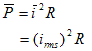If we pass direct current of magnitude irms through the resistor ,the power dissipate or rate of production of heat in this case would be
P=(irms)2R
• Thus rms value of AC is that value of steady current which would dissipate the same amount of power in a given resistance in a given tine as would gave been dissipated by alternating current
• This is why rms value of AC is also known as virtual value of current

## (5) Phasor diagram

• Phasor diagrams are diagram representing alternating current and voltage of same frequency as vectors or phasors with the phase angle between them
• Phasors are the arrows rotating in the anti-clockwise direction i.e. they are rotating vectors but they represents scalar quantities
• Thus a sinusoidal alternating current and voltage can be represented by anticlockwise rotating vectors if they satisfy following conditions
• Length of the vector must be equal to the peak value of alternating voltage or current
• Vector representing alternating current and voltage would be at horizontal position at the instant when alternating quantity is zero
• In certain circuits when current reaches its maximum value after emf becomes maximum then current is said to lag behind emf
• When current reaches its maximum value before emf reaches its maximum then current is said to lead the emf
• Figure below shows the current lagging behind the emf by 900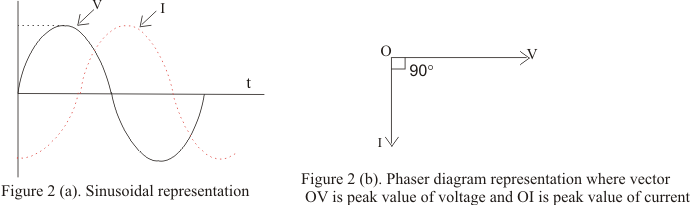## (6) A.C through pure resistor

• Figure below shows the circuit containing alternating voltage source V=V0sinω connected to a resistor of resistance R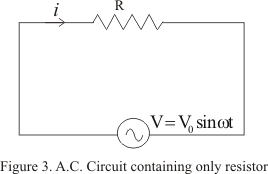• Let at any instant of time ,i is the current in the circuit ,then from Kirchhoff�s loop rule
V0sinωtRi
or
i=(V0/R)sinωt
=i0sinωt                                                ----(8)
Where,
i0=V0/R                                                ----(9)
• From instantaneous values of alternating voltage and current ,we can conclude that in pure resistor ,the current is always in phase with applied voltage
• Their relationship is graphically represented as